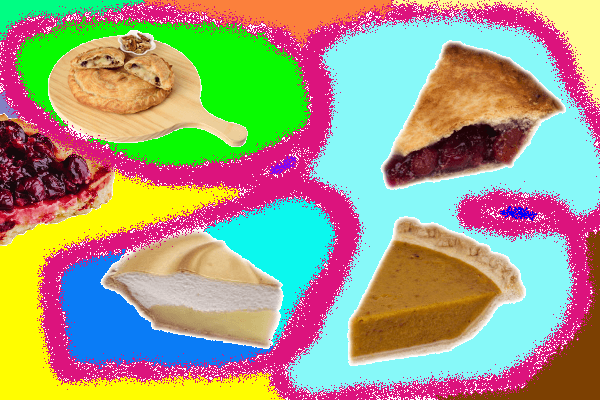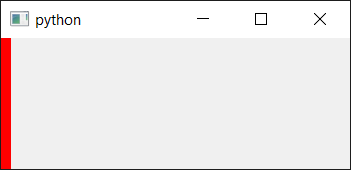# Implementing QPainter flood fill in PyQt5/PySide Filling irregular regions in a QPainter canvas

Building Piecasso (a PyQt5 Paint clone) I was disappointed to discover that while QPainter comes with a huge number of paint methods, ranging from pixels and lines to fully-filled polygons, it doesn't include a method for flood filling regions of an image.

That makes a lot of sense, firstly because flood-filling is slow to do — requiring a pixel-by-pixel search through an image — and it's not that useful when drawing a UI, since you usually (and probably should) know what you're drawing and where.

Still, I was disappointed there wasn't one, because I needed one for my app. What's Paint without being able to fill raggedy shapes in horrible colours?Raggedy fill

In this short walkthrough I'll cover the process of implementing a basic flood-fill algorithm in PyQt5, using `QImage.pixel()`. If you find yourself needing flood fill in your own apps, this will do the trick.

## Flood fill algorithm

The implementation here uses a basic Forest Fire flood fill algorithm. In this approach we start from any given pixel, and iteratively check if any adjacent pixels match, and then any adjacent pixels of those pixels, and …so on. Once we've tested a given pixel we don't need to return to it so we can colour it with our target colour.

This is analogous to a forest fire which starts from a given point and spreads outwards, but will not return to areas it's already "burnt" (changed colour). The following animation gives a good visualisation of the process.The steps of the algorithm are explained below.

1. Start with our start pixel, fill colour, and two empty lists `seen` and `queue`.
2. Look at our current pixel's colour. This is the target for our fill. Store this initial location in `queue`.
3. Taking the first item from `queue` (initially our start (x,y) location) look at each of the 4 pixels surrounding our location (cardinal points)
1. If they have not been previously seen — compare their colour to the one we're looking for.
4. If they match, add the (x,y) location to `queue` and update the pixel with the fill colour.
5. Add the (x,y) location to `seen` to keep track of where we've looked before (and avoid the overhead of looking again).
6. Repeat from step 3, until the queue is empty.

You can opt to check 8 directions, if you want to be able to fill through diagonal gaps.

The order you visit pixels will change depending on whether you add new locations to the beginning or end of your `queue` list. But this won't affect the result.

Below we'll look at implementing this algorithm in Python, using PyQt5/PySide2. The `fill` methods described below can each be inserted into the following app skeleton if you want to test them yourself.

python
``````from PyQt5 import QtCore, QtGui, QtWidgets

from PyQt5.QtGui import QPainter, QPen, QColor
from PyQt5.QtCore import QPoint

FILL_COLOR = '#ff0000'

class Window(QtWidgets.QLabel):

def __init__(self, *args, **kwargs):
super(Window, self).__init__(*args, **kwargs)

p = QtGui.QPixmap(dim, 500)
p.fill(QtGui.QColor('#ffffff')) # Fill entire canvas.
self.setPixmap(p)

self.fill(0, 0)

def fill(x, y):
# ... see below ..

app = QtWidgets.QApplication(sys.argv)
w = Window()
w.show()
app.exec_()
``````
python
``````from PySide2 import QtCore, QtGui, QtWidgets

from PySide2.QtGui import QPainter, QPen, QColor
from PySide2.QtCore import QPoint

FILL_COLOR = '#ff0000'

class Window(QtWidgets.QLabel):

def __init__(self, *args, **kwargs):
super(Window, self).__init__(*args, **kwargs)

p = QtGui.QPixmap(dim, 500)
p.fill(QtGui.QColor('#ffffff')) # Fill entire canvas.
self.setPixmap(p)

self.fill(0, 0)

def fill(x, y):
# ... see below ..

app = QtWidgets.QApplication(sys.argv)
w = Window()
w.show()
app.exec_()
``````

## `QImage.pixel()`

To identify which pixels are filled with the correct colour we can use `QImage.pixel()`. This accepts an `x` and `y` coordinate and returns the colour at the given coordinates. There are two methods available — one to return the colour of the pixel as `QRgb` object, one as a `QColor`.

python
``````QImage.pixel(x, y)       # returns a QRgb object
QImage.pixelColor(x, y)  # returns a QColor object
``````

Below is an implementation of the fill algorithm described above using direct pixel access via `QImage.pixel`. We'll use this to generate some timings.

python
``````image = self.pixmap().toImage()
w, h = image.width(), image.height()

# Get our target color from origin.
target_color = image.pixel(x,y)

have_seen = set()
queue = [(x, y)]

def get_cardinal_points(have_seen, center_pos):
points = []
cx, cy = center_pos
for x, y in [(1, 0), (0, 1), (-1, 0), (0, -1)]:
xx, yy = cx + x, cy + y
if (xx >= 0 and xx < w and
yy >= 0 and yy < h and
(xx, yy) not in have_seen):

points.append((xx, yy))

return points

# Now perform the search and fill.
p = QPainter(self.pixmap())
p.setPen(QColor(FILL_COLOR))

while queue:
x, y = queue.pop()
if image.pixel(x, y) == target_color:
p.drawPoint(QPoint(x, y))
# Prepend to the queue
queue[0:0] = get_cardinal_points(have_seen, (x, y))
# or append,
# queue.extend(get_cardinal_points(have_seen, (x, y))

self.update()
``````

This code fragment will work as-is on a `QLabel` widget which is displaying a `QPixmap` image (returned by `self.pixmap()`). However you can modify it to work on any `QPixmap`.

First we get an image representation of the `QPixmap`, so we can perform the `.pixel()` lookup operation. Then we get the dimensions, to determine the limits of our search, and finally, the target colour — taken from the pixel where we start the fill.

python
``````image = self.pixmap().toImage()
w, h = image.width(), image.height()

# Get our target color from origin.
target_color = image.pixel(x,y)

have_seen = set()
queue = [(x, y)]
``````

The `set()` named `have_seen` is used to track pixels that we've already visited, and therefore do not need to revisit. This is technically not necessary since pixels we've visited will be recolored and no longer match the original point, but it's quicker.

The `queue` holds a list of `(x, y)` tuples of all locations that we still need to visit. The `queue` is set to our initial fill position, and `have_seen` is reset to an empty set.

To determine what to put in the queue, we call the method `get_cardinal_points` which for a given position looks at all surrounding positions — if they haven't been looked at yet — and tests whether it is a hit or a miss.

python
``````def get_cardinal_points(have_seen, center_pos):
points = []
cx, cy = center_pos
for x, y in [(1, 0), (0, 1), (-1, 0), (0, -1)]:
xx, yy = cx + x, cy + y
if (xx >= 0 and xx < w and
yy >= 0 and yy < h and
(xx, yy) not in have_seen):

points.append((xx, yy))

return points
``````

If it's a hit, we return that pixel to look and fill later. The result of this method is added to the `queue` in the main loop of the search.

python
``````while queue:
x, y = queue.pop()
if image.pixel(x, y) == target_color:
p.drawPoint(QPoint(x, y))
# Prepend to the queue
queue[0:0] = get_cardinal_points(have_seen, (x, y))
``````

This loops over the current queue, which is constantly expanding. Each loop it removes the first element `x` and `y` positions, checking the pixel at that location. If it is a match, we draw our fill colour. Finally, we check all cardinal points of the current location, updating the `queue` and `have_seen` as appropriate.

Below is a table showing the time taken to fill an area of the given size (in pixels) using this algorithm.

x y Area Time (s)
500 10 5000 0.0145067
500 50 25000 0.0726196
500 100 50000 0.1422842
500 500 250000 0.7466553
500 1000 500000 1.5315427
500 5000 2500000 7.8900651

As you can see, at smaller fill areas the speed is reasonable, later it gets quite slow. If you run the code, you'll see the following image only (the final 500x10 run).Flood fill result at 500x10 pixels

You can opt to save the images on each iteration if you like, in order to check they are working.

python
``````self.pixmap().save(<filename>)
``````

Filling an area of 500 x 100 pixels takes > 100 ms while a 500 x 5000 pixel region takes almost 8 seconds. So don't use flood fills in parts of your application that are performance-dependent, such as when drawing custom widgets.

Over 10,000 developers have bought Create GUI Applications with Python & Qt!
[[ discount.discount_pc ]]% OFF for the next [[ discount.duration ]] [[discount.description ]] with the code [[ discount.coupon_code ]]